# How to use subtraction in Google Sheets

Calculations in Google Sheets will make it easier for you to calculate numbers, instead of manual calculations similar to subtraction in Excel. To perform subtraction in Google Sheets, we can use functions in Google Sheets, or use the minus sign in Google Sheets to use. The following article will guide you through ways to subtract in Google Sheets.

## Use the minus MINUS function in Google Sheets

The MINUS function in Google Sheets will quickly subtract 2 numbers together, giving you faster results. The function formula is =MINUS(value 1, value 2).

With the values ​​below, you just need to enter the value for the subtraction calculation and press Enter to display the results.

To subtract values ​​in cell like B2 minus B6 then you also enter the corresponding cell value as below. Then we press Enter and also get the result of the subtraction.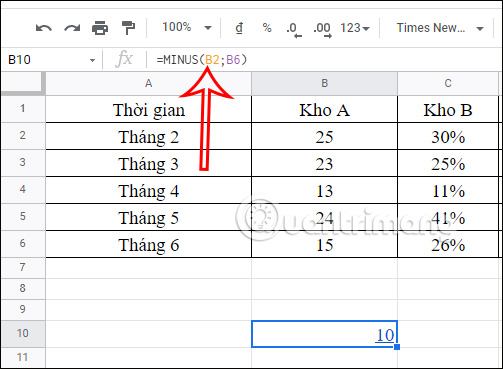## How to use the – in Excel

Besides using the MINUS function in Google Sheets to subtract, we can also use the minus sign to subtract values ​​from each other.

It’s very simple, you just need to enter the value of the calculation to be subtracted.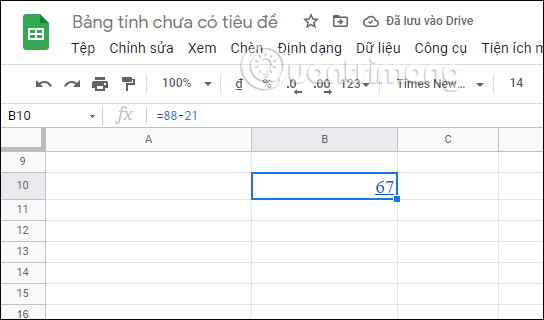With cell value subtraction, you also enter the cell value and press Enter to get the result of the subtraction.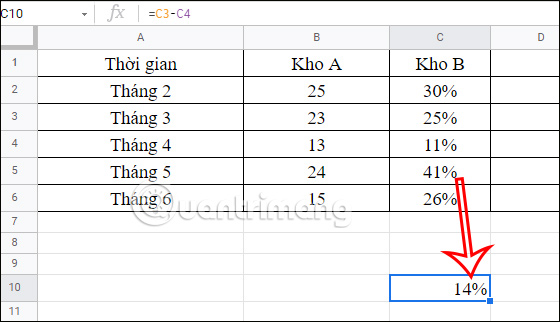## How to use the SUM function in Google Sheets

In addition to using the SUM function to add values ​​in Sheets, we can also use the SUM function to subtract in Google Sheets.

We will enter the formula as shown below to subtract in Google Sheets.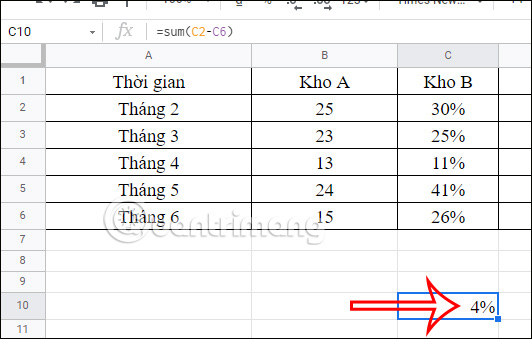## How to create a calculation expression in Sheets

Besides performing simple calculations, you can completely create expressions for addition, subtraction, multiplication and division.

The subtraction with multiplication has a cell value, then you will enter the formula as shown below. Then we get the result for the calculation.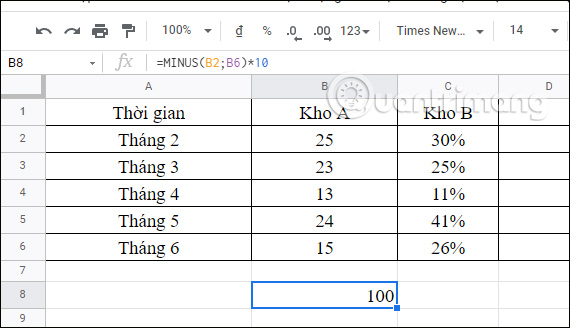Similarly you can also create addition and subtraction with numbers in Sheet as shown below.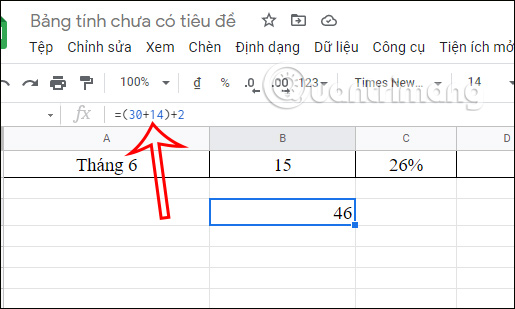With the SUM function, you can also create a calculation expression as shown. We’ll sum the range and then subtract some value.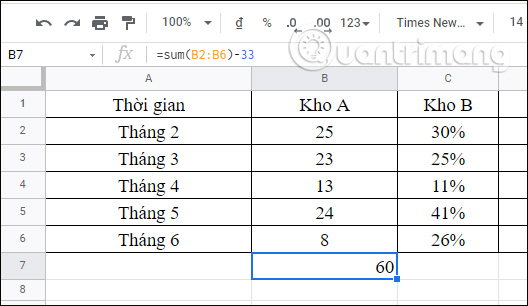.